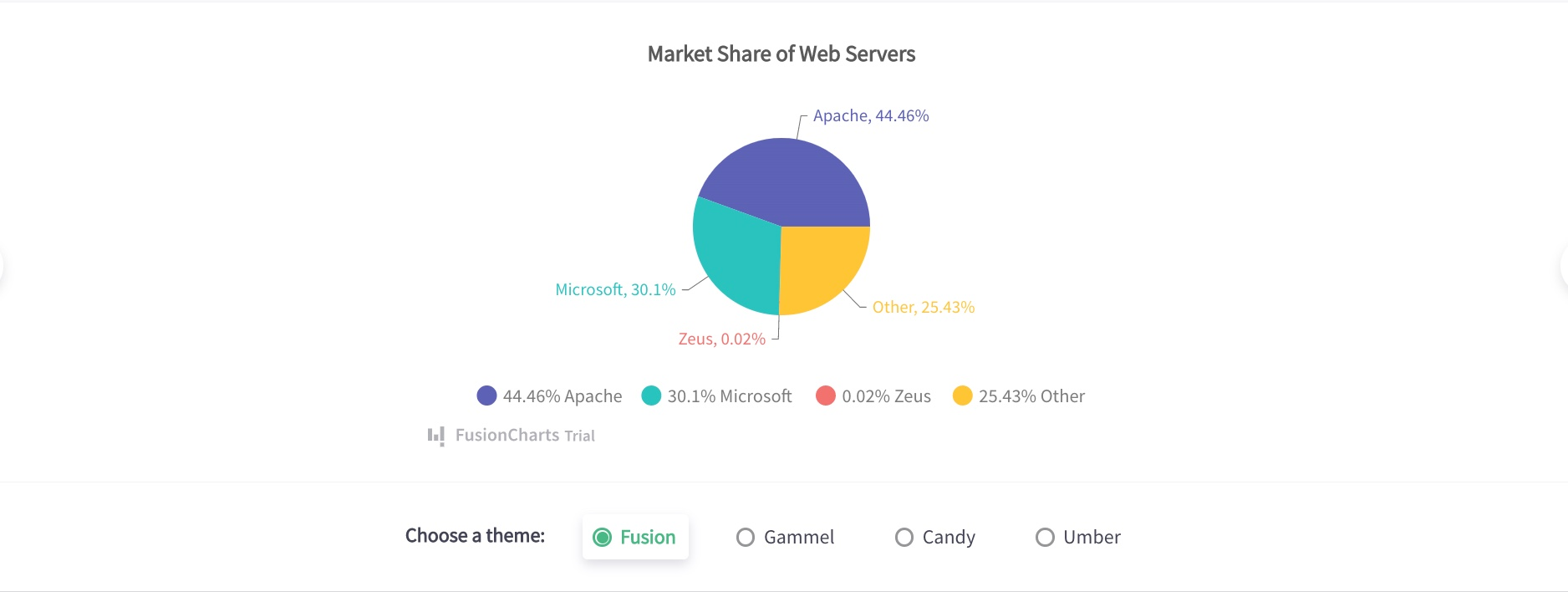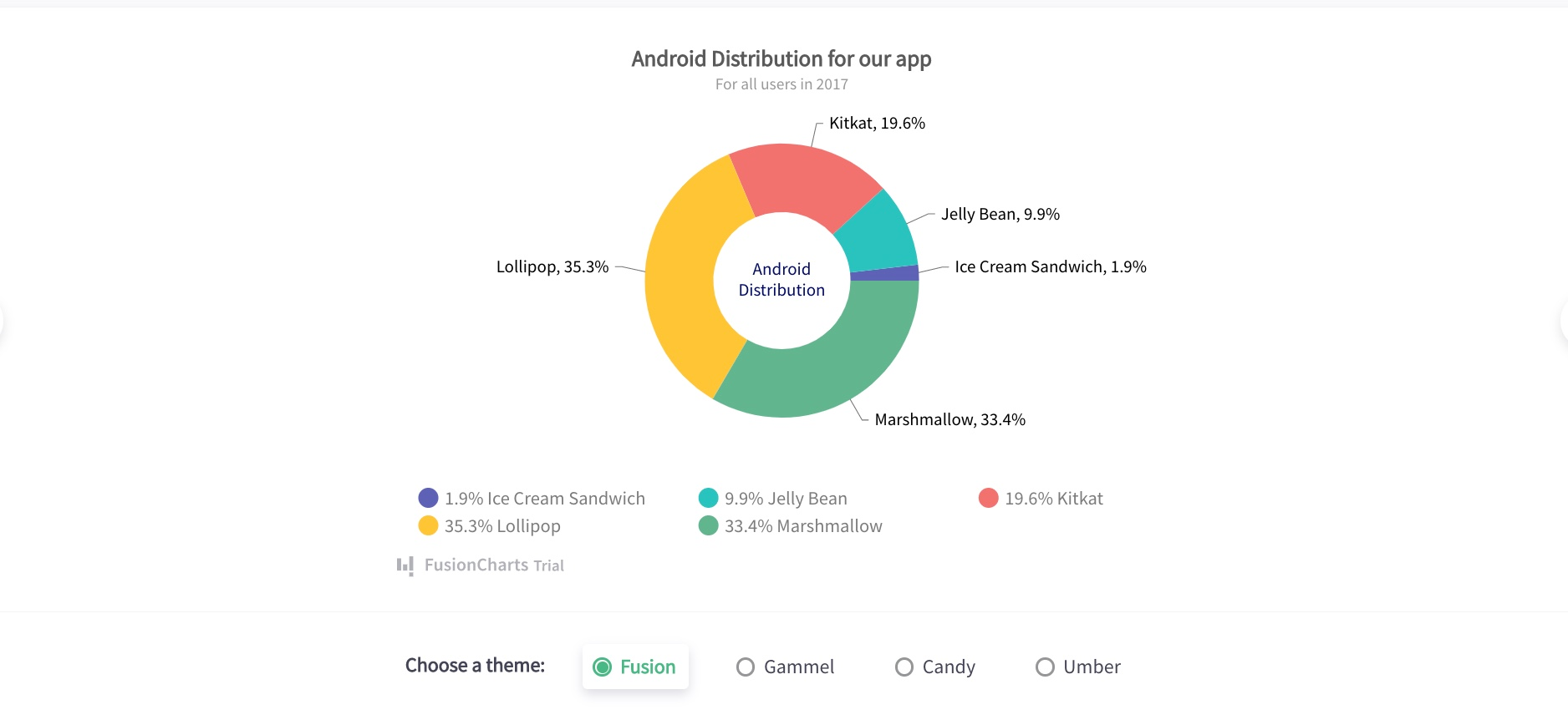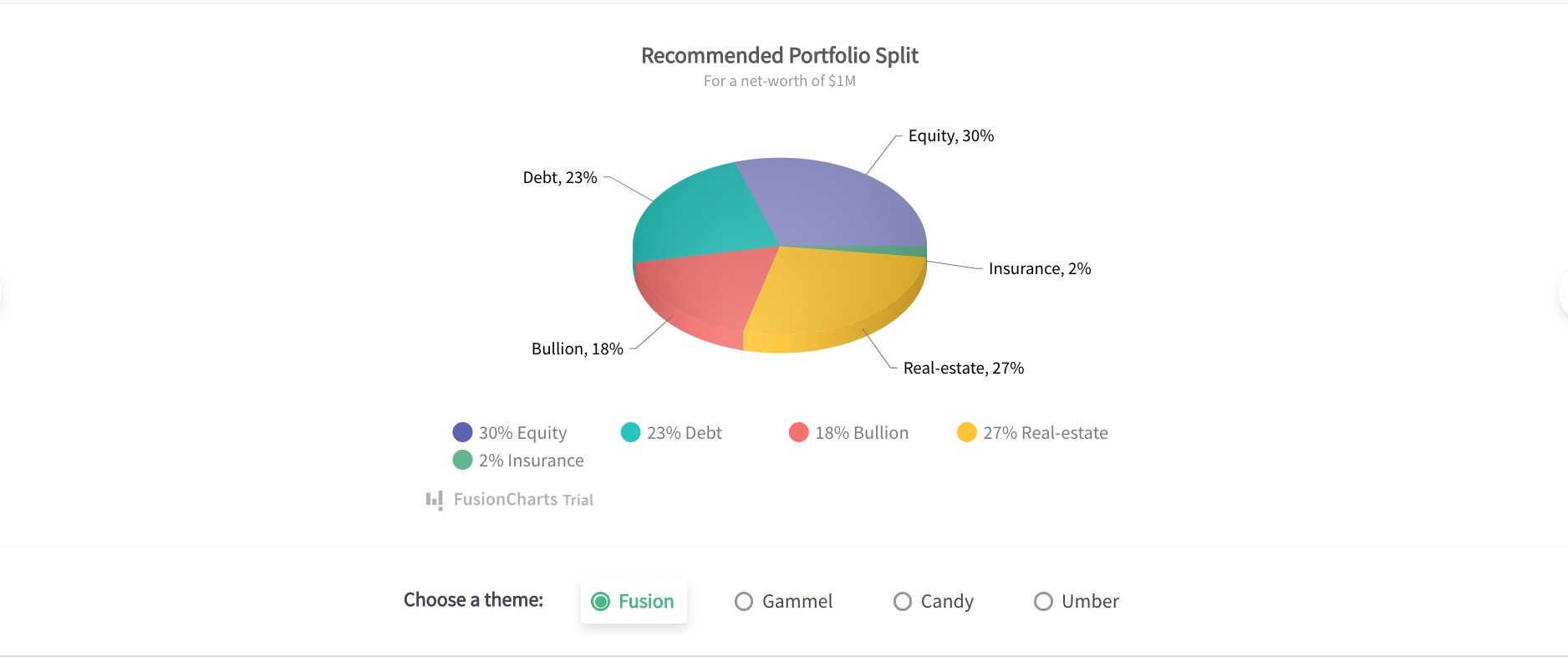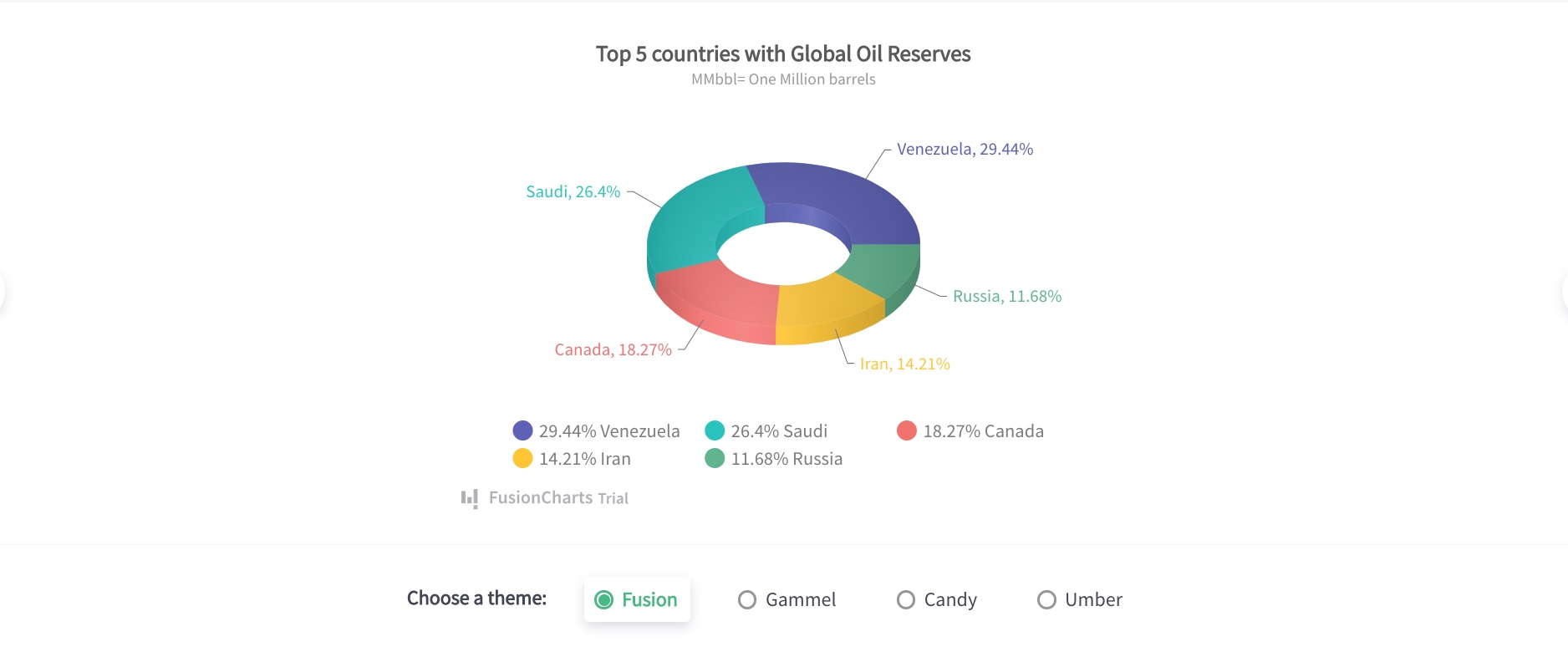Pie charts are suited for comparing multiple categories or showing part-to-whole relationships in a single data set. The circular-shaped graphs are easy to create (using pie chart makers) and are used in a variety of industries. In this post, we’ll learn about real-life use cases, pie chart examples with explanations, and some limitations you should have in mind.

## What Is A Pie Chart?

A pie chart is a circular-shaped chart that visually demonstrates the part-whole relationship of categorical data variables. The circular chart is rendered as a circle that represents the total amount of data while having slices that represent the categories. Each slice in the pie visualizes each variable (or category), with the arc size and length showing its quantity.

## Uses Of Pie Chart

Pie charts are essential for data visualization when you’re working with varied data sets and need to compare part to whole. Plus, these charts are also pretty easy to interpret and can be utilized in a variety of fields and industries. Here are a few uses of pie charts. Sales Pie charts are relevant in analyzing sales data. For instance, accountants use them to compare the quantity of sales for various products in a store or profit growth for a business in different months. School Pie charts are utilized in schools for various purposes. For example, teachers use them to visualize the time for each lesson period or show how many students scored each grade in a test. Marketing Marketers use pie charts to visualize the breakdown of Ad spend across various marketing channels.

## Examples Of Pie Charts

Here are some pie chart examples and common use cases in different industries.

### Example 1

The 2D pie chart is a simple circle divided into sectors to represent each category in a dataset. We use this type of pie chart to visually compare the share of categories as part of the whole. The example below shows a 2D pie chart that visually depicts the market share of web servers: Apache (44.46%), Microsoft (30.1%), Zeus (0.02%), and Others (25.43%). To determine the degrees of each category from the given data, we will use the equation: (Category % / 100) * 360. Here is how the pie chart appears.### Example 2

Doughnut charts are another variation of a 2D pie chart and have a space at the center to look like a doughnut. We use them to depict part-whole relationships. This pie chart example below is a pictorial representation of the Android distribution for an app using this given data (in percentage of the total value): Lollipop (35.3%), Marshmallow (33.4%), KitKat (19.6%), Jelly Bean (9.9%), and Ice Cream Sandwich (1.9%). Given the information and summary statistic above, we can determine the angle (degrees) of each category using the equation: (Category % / 100) * 360.### Example 3

3D Pie charts function like regular 2D pie charts but possess some depth to each slice and a layer of aesthetics. We use them to depict the difference in relative sizes between categories or the percentage split of the total data value. Below, you’ll find a 3D pie chart example that visually illustrates the recommended portfolio split for a net worth of \$1M. Given all the values for the chart: Equity (30%), Real Estate (27%), Debt (23%), Bullion (18%), and Insurance (2%), we can calculate how many degrees is to be constructed for each category using the equation: (Category % / 100) * 360. Plus, we can also calculate the actual amount (\$) for each category using the equation: (Category % / 100) * \$1M. Here is the pie chart example for the same data above.### Example 4

3D Donut charts are a variation of doughnut graphs that contain a layer of aesthetics. Like the 2D variants, we also use them for data comparison and to illustrate the proportional data of various categories. The pie chart example below shows the top 5 countries with global oil reserves. The given data in percentage for each pie sector include Venezuela (29.44%), Saudi (26.4%), Canada (18.27%), Iran (14.21%), and Russia (11.68%). With the categorical variables above, we can get the relative size of oil reserves (million barrels) for each country using the equation: (Country  % / 100) * 1MMbbl. And before creating a pie chart in react native, we can determine the degree angle for each country using the equation: (Category % / 100) * 360.## Tips For Understanding Pie Chart Examples

Here are some major tips to understand and consider when you want to create pie charts.

### Avoid negative values

You should avoid using negative values in all the data when you draw your pie chart. Doing this will ensure your pie chart is not affected and your audience isn’t confused during interpretation.

### Minimize the number of sections

Having plenty of slices makes the chart cumbersome to understand and is one of the few pie chart disadvantages. It is advisable to draw a minimum number of categories on the circular graph. An ideal range is between 4 and 7 slices.

### Use different colors

Slices in pie charts should be distinguished with unique colors. This would make it easy for your audience to differentiate between categories.

### Display nominal & original data

Before deciding on using a pie chart, ensure the data categories you need to visualize are nominal and ordinal.

## Limitations Of Pie Charts

Pie charts are perfect in cases where you have a single data set and want to compare categories to the whole. Hence, they are not suitable for every type of statistical or presentation data. Here are some limitations you should have in mind when employing a pie chart:

### High number of categories

Pie charts can’t properly handle a large number of numerical variables. If the slices in the pie go above 6 or 7, the chart becomes crowded and challenging to read. Hence, you should explore other types of charts in this situation and consider the advantages of using bar graphs vs pie charts for instance.

### Categories with equal sizes

If most or all categories in your data possess approximately equal sizes, you can’t make visual comparisons with ease and quickly understand information by simply looking at the pie chart. In this situation, it is preferable to consider using other types of charts such as bar charts.

### Comparison of multiple data sets

It is impossible to create a pie chart to measure two sets of data. To visually compare two data sets, you should create a single bar chart for visual representation.

Let’s take a look at some commonly asked questions about pie chart examples.

### Why do we use pie charts?

We use pie charts to mostly visualize the categories as a proportion of the total value. In a single chart, you’ll find pie slices that represent the percentage of a particular data from the whole pie.

### How do you explain a pie chart?

We can describe a pie chart can as a full circle divided into areas called slices. Each pie slice in the circle represents a category and the values of each slice add up to the total. Pie charts are popular in data visualization as a communication tool.

### How do you calculate a pie chart?

To calculate the degree for each category, divide the category amount by the total amount and then multiply by 360. Each degree that we obtain for each category is used to create a pie chart.

### What are the 2 types of pie charts?

Based on graph dimension, we can group pie charts into two main types namely: 2D pie charts and 3D pie charts.

## Conclusion

In summary, pie charts are notable for part-to-whole comparisons. They are used in various industries: education, oil and gas, business, health, and even technology. Creating a pie chart is simple and straightforward. The first step is to compile all the data needed, and convert them to degrees (and percentages). Then, construct each slice appropriately and add data labels. From the examples in this post, you can clearly see how we apply pie charts as an effective communication tool in real-life scenarios. If you’re looking to start replicating any of these pie chart examples, you can sign up for a free trial with FusionChart today.

## Take your data visualization to a whole new level

From column to donut and radar to gantt, FusionCharts provides with over 100+ interactive charts & 2,000+ data-driven maps to make your dashboards and reports more insightful

This site uses Akismet to reduce spam. Learn how your comment data is processed.

##### Your next great dashboard starts here

With our interactive and responsive charts, extensive documentation, consistent API, and cross-browser support - delight your customers with kick-ass dashboards